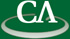## Sparse techniques to speed up multivariate polynomial factorization.

### Wednesday July 17th at 3:00pm in K9509.

```Abstract

The standard approach to factor a multivariate polynomial
in Z[x1,x2,...,xn] is to factor a univariate image in Z[x1]
then recover the multivariate factors from their images using
a process known as multivariate Hensel lifting.
For the case when the factors are expected to be sparse,
at CASC 2016, we introduced a new approach which uses
sparse polynomial interpolation to solve the multivariate
polynomial diophantine equations that arise inside Hensel lifting.

In this work we extend our previous work to the case when the
number of factors to be computed is more than 2.   Secondly,
for the case where the integer coefficients of the factors are
large we develop an efficient p-adic method.
We will argue that the probabilistic sparse interpolation method
introduced by us provides new options to speed up the factorization
for these two cases.  Finally we present some experimental
data comparing our new methods with previous methods.

This is joint work with Baris Tuncer.

```